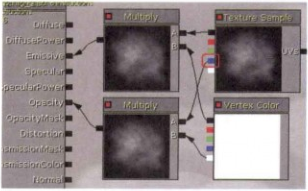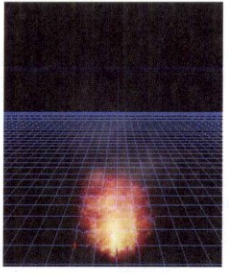# UDK游戏引擎 “火焰”特效制作教程（二）制作烟雾效果与火星

3.制作“烟雾

step01 为了使火焰效果更真实，在火焰的上方添加烟雾效果。在[内容浏览器]窗口中选择“T_FX1”贴图，建立名为"smoke" 的材质，添加节点制作粒子材质，为了使“烟雾”效果更透明，将贴图的蓝色通道节点连接到Multiply (乘)节点上作为透明通道，如图所示。step02 设置材质的Blend Mode (混合模式) 为“BLEND_ Additive" ，Lighting Model (光照模型)为“MLM_Unlit" 。

step03 在“Flames"粒子发射器上单击鼠标右键，选择[发射器]|[克隆发射器]选项，将克隆的粒子发射器更名为“Smoke” ， 将前面创建的材质添加到Required (必要)的Material (材质)中。

step04 为了使“烟雾”高于“火苗”，修改Lifetime (生命周期)属性，设置其Distribution (分布)的值，[Min] 为0.8，[Max] 为3。

step05 修改“烟雾” 颜色，设置[ Color Over Life (随生 命周期改变颜色) ]数值， 单击夏[添加新项目]按钮，设置项中[In Val]的值为0，[Out Val]的值为(X=3,Y=1,Z=1) ; 项中[InVal]的值为0.5，[Out Val]的值为(X=1,Y=1,Z=1) ;  项中[In Val]的值为1，[Out Val]的值为(X=0.2,Y=0.2,Z=0.2)，调整其动画曲线，使粒子随时间变化，颜色也产生变化。

step06 设置“烟雾” 速度，使其速度比 “火苗”速度稍慢，设置[ Initial Velocity ]中[ Distribution ]的[Max]值为(X=10,Y=10,Z=30)，[Min] 的值为(X-=-10,Y=-10,Z: *20)，如图所示。4.制作“火星”

step01 在“Flames" 粒子发射器上单击鼠标右键，选择[发射器]|[克隆发射器]选项,将克隆的粒子发射器更名为“Star”。

step02修改[Spawn (繁殖) ]属性，设置其[Distribution] 的[Rate (速率) ]值为6。

step03 修改[ Lifetime (生命周期) ]属性， 设置其[ Distribution] 的[Min] 值为0.8， [ Max ]的值为3。

step04 修改[ Initial Size (初始大小) ]属性，设置其[ Distribution] 的[ Max]值为 (X=1.5,Y=1.5,Z=1.5)，[Min]的值为(X=1,Y=1,Z=1 )。

step05 修改[ Initial Velocity (初始速度) ]属性，设置其[ Distribution]的[Max]值为(X=10,Y=10,Z=30) ，[Min] 的值为(X-=-10,Y=-10,z=20) 。